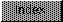```

NAME
afp - Convert ASCII string variable into fast floating point

SYNOPSIS
ffp_value = afp(string);

FUNCTION
Accepts the address of the ASCII string in C format that is
converted into an FFP floating point number.

The string is expected in this Format:
{S}{digits}{'.'}{digits}{'E'}{S}{digits}
<*******MANTISSA*******><***EXPONENT***>

Syntax rules:
Both signs are optional and are '+' or '-'. The mantissa must be
present. The exponent need not be present. The mantissa may lead
with a decimal point. The mantissa need not have a decimal point.
Examples: All of these values represent the number fourty-two.
42            .042e3
42.          +.042e+03
+42.          0.000042e6
0000042.00       420000e-4
420000.00e-0004

Floating point range:
Fast floating point supports the value zero and non-zero values
within the following bounds -
18                             20
9.22337177 x 10   > +number >  5.42101070 x 10
18                             -20
-9.22337177 x 10   > -number > -2.71050535 x 10

Precision:
This conversion results in a 24 bit precision with guaranteed
error less than or equal to one-half least significant bit.

INPUTS
string - Pointer to the ASCII string to be converted.

OUTPUTS
string - points to the character which terminated the scan
equ - fast floating point equivalent

```